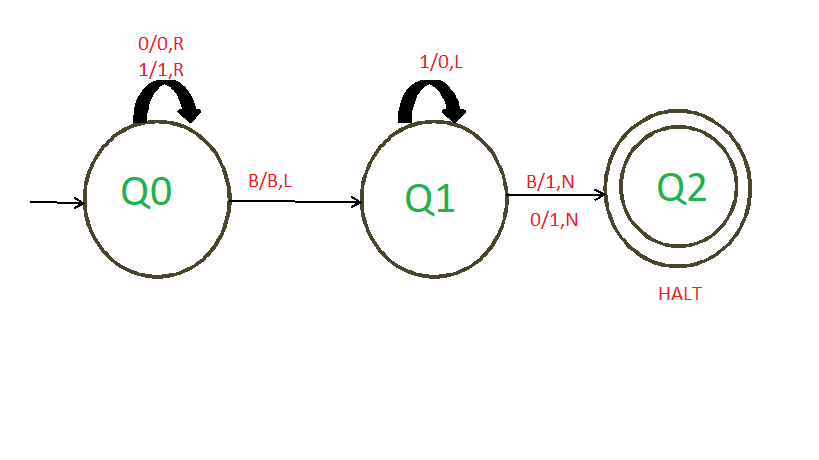# Construct Turing Machine for incrementing Binary Number by 1

• Difficulty Level : Basic
• Last Updated : 29 Sep, 2020

Prerequisite : Turing Machine

We have to design a Turing Machine for incrementing the Binary Number by 1.

Examples –

```Input: 10111
Output: 11000

Input: 1000
Output: 1001

Input: 10101011
Output: 10101100```

Analysis :
From the above three examples, we can get two conditions –

• When the Rightmost digit is 0 :
Here we can see that when we add something to a binary number having 0 as its rightmost digit, then the rightmost digit of the Binary number changes i.e. if the rightmost digit is 0 it will change to 1 and vice versa while all other digits remain the same and our machine will halt when we get Blank(B).

• When the Rightmost digit is 1 :
Here we can see that when we add something to a binary number having 1 as its rightmost digit, then all the 1’s changes to 0’s until we get a 0, and the 0 we get will change to 1 while all other digits after that will remain same and our machine will halt when we get Blank(B). Suppose we don’t have any 0 in a string for example 1111 then we move to the left until we get a Blank(B) changing all the 1’s to 0’s and change this Blank(B) to 1, and our machine halts.

Approach :

1. We have to scan the element from right to left. At first, our pointer is at the leftmost side. Therefore we have to shift the pointer to the rightmost side.
2. To shift the pointer to the rightmost side we normally skip all 0’s and 1’s until we get a Blank(B).
3. After this step, we can now move the pointer from left to right.
4. If we get the first digit 1 then we change all the 1’s to 0’s until we get a 0 and change this 0 to 1. After this, all the digit remains the same, and our machine halts at Blank(B).
5. If we get the first digit 1 then a condition arises that we don’t get any 0 for example 1111, then we move to the left until we get a Blank(B) changing all the 1’s to 0’s and change this Blank(B) to 1, and our machine halts.
6. If we get the first digit as 0 then we have to change 0 as 1 and after that, all the digit remains the same and our machine will halt at Blank(B).My Personal Notes arrow_drop_up